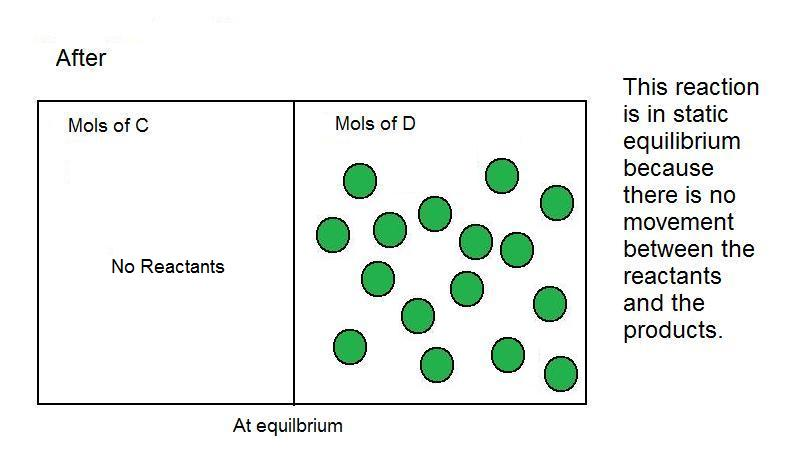# Dynamic equilibrium

At dynamic equilibrium, the reaction rate of the forward reaction is equal to the reaction rate of the backward reaction.

## Introduction

A reaction rate determines how fast a reaction proceeds, and is mathematically defined as the change in concentration of a species over the change in time. For more information, please read Measuring Reaction Rates. An example of a fast reaction would be fire burning (combustion of oxygen) and an example of a slow reaction is iron rusting (oxidation of a metal). Reactions do not only proceed forward; reactions often occur in a mixture of forward and reverse, the more prevalent being the observed course of the reaction.

Reactions should not be conceptualized as one substance completely changing into another; rather, they should be visualized as having two components acting in opposite directions. In this scenario, there is a point where the two components are acting at equal rates; this is referred to as equilibrium. At equilibrium, the reaction rates of the forward and reverse reactions are equal. There are two different types of equilibrium: dynamic equilibrium and static equilibrium.

## Dynamic Equilibrium

At dynamic equilibrium, reactants are converted to products and products are converted to reactants at an equal and constant rate. Reactions do not necessarily—and most often do not—end up with equal concentrations. Equilibrium is the state of equal, opposite rates, not equal concentrations.

## Static Equilibrium

Static equilibrium, also called mechanical equilibrium, occurs when all particles in the reaction are at rest and there is no motion between reactants and products. Static equilibrium can also be seen as a steady-state system in a physics-based view. Dynamic forces are not acting on the potential energies of the reverse and forward reactions. An example of static equilibrium is graphite turning into diamond, shown below. This reaction is considered at static equilibrium after it occurs because there are no more forces acting upon the reactants (graphite) and products (diamond).

$C(graphite) \rightarrow C(diamond)$

Consider the following general reaction:

$C \rightarrow D$

Figure 3: Before the reaction of C to D occursFigure 4: Reaction C--> D at static equilibrium under equilibrium conditionsNote: Static equilibrium does not necessarily mean there are no moles on the reactant side after the reaction has occurred. This is only an example of static equilibrium.

## Le Chatelier's Principle and Equilibrium

Equilibrium can be explained by Le Chatelier's Principle. The kinetics of a reaction change and position of equilibrium depend on the properties of the reactants and products. Equilibrium shifts towards one side or the other depending on concentration, temperature, pressure, and volume. Le Chatelier's principle is not the same as dynamic equilibrium; they are similar but distinct concepts. Le Chatelier's principle describes how equilibrium can change. Dynamic and static equilibrium describe how equilibrium behaves.

## Comparison between Dynamic and Static Equilibrium

A reaction at dynamic equilibrium can be reversible, whereas a reaction at static equilibrium is irreversible. A reversible reaction is a reaction that can proceed in the direction of products to reactants. The equilibrium constant does not indicate whether a reaction is in static or dynamic equilibrium because it is simply the concentrations of the products divided by the concentrations of the reactants. A reaction is at dynamic equilibrium if the rate of the forward reaction is equal to the rate of the reverse reaction. It is at static equilibrium if the reaction has occurred and the forward and reaction rates are both equal to 0. The rate of reactions cannot be determined by the stoichiometric factors of a balanced equation; reaction orders and reaction rates must be determined experimentally.

## Relationship Between Equilibrium and Rate Constants

Consider the following simple reaction:

$A \rightleftharpoons B$

where A denotes the reactants and B denotes the products. The equilibrium constant, Keq, is defined as:

$K_{eq}= \dfrac{[B]_{eq}}{[A]_{eq}}$

where [A]eq represents the reactants at equilibrium conditions and [B]eq represents the products at equilibrium conditions. The rate of the reaction is given by:

$\dfrac{d[A]}{dt}= -k_f[A]+k_b[B]$

where kf is the rate constant for the forward reaction and kb is the rate constant for the backward reaction. The equilibrium constant can also be calculated by dividing the rate constant of the forward reaction by the rate constant of the reverse reaction:

$K_{eq}=\dfrac{k_f}{k_b}$

where A and B are in equilibrium.

## References

1. Petrucci, Harwood, Herring, and Madura. General Chemistry: Principles and Modern Applications. 9th ed. Upper Saddle River, New Jersey: Pearson Education, 2007.
2. Zhumdal, Zhumdal. Chemistry. 7th ed. Boston, New Jersey: Massachusetts Houghton Miffle Company, 2007.